# Texas Go Math Grade 1 Lesson 5.4 Answer Key Use Pictures and Subtraction to Compare

Refer to our Texas Go Math Grade 1 Answer Key Pdf to score good marks in the exams. Test yourself by practicing the problems from Texas Go Math Grade 1 Lesson 5.4 Answer Key Use Pictures and Subtraction to Compare.

## Texas Go Math Grade 1 Lesson 5.4 Answer Key Use Pictures and Subtraction to Compare

Essential Question
How can you use pictures to compare and subtract?
Explanation:
Comparing by one to one
By using the pictures we have to compare 1 to 1

Draw bowls to show the problems. Draw lines to match.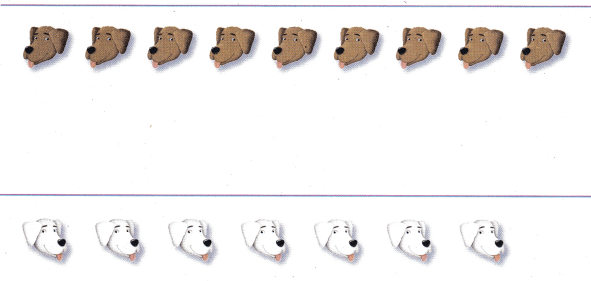Explanation: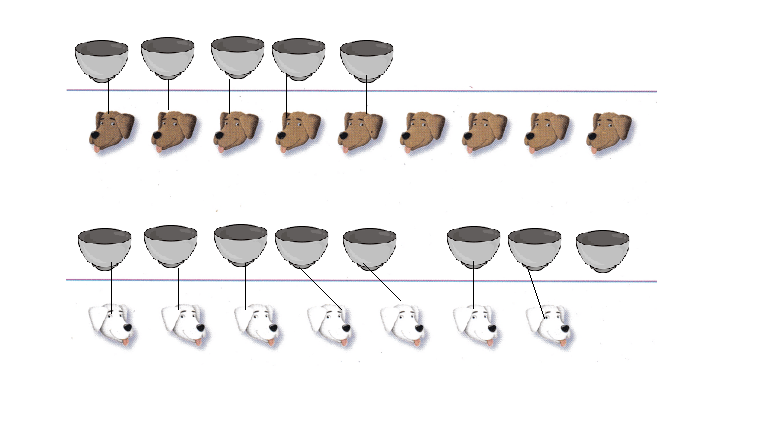4 More Brown dogs than bowls
1 more bowl than white dog

Math Talk
Mathematical Processes
Explain how many more dogs or bowls there are.
Explanation:
4 More Brown dogs than bowls
1 more bowl than white dog

For The Teacher

• Read the problem. There are 9 brown dogs. There are 5 bowls. How many more dogs need a bowl? There are 7 white dogs. There are 8 bowls. How many bowls are not needed?4 More Brown dogs than bowls
1 more bowl than white dog

Model and Draw

Compare the groups. Subtract to find how many fewer or how many more.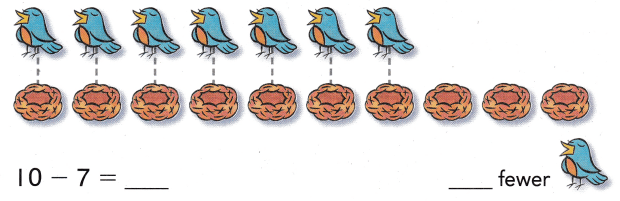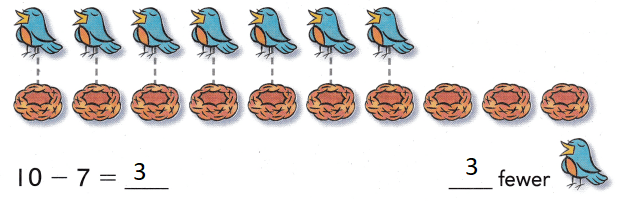Explanation:
10 – 7 = 3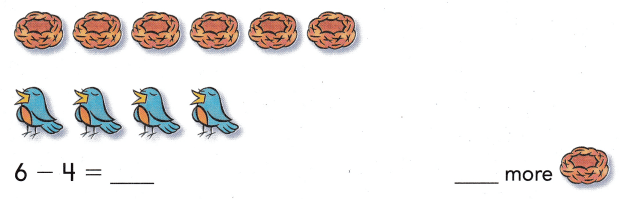Explanation:
6 – 4 = 2
2 more food

Share and Show
Draw lines to match. Subtract to compare.

Question 1.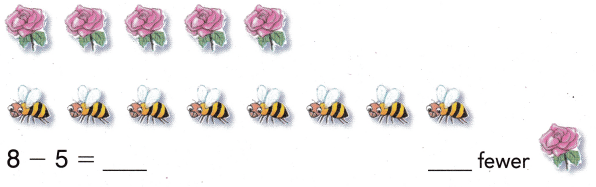Explanation:
8 – 5= 3
3 fewer bees

Problem Solving

Draw lines to match. Subtract to compare.

Question 2.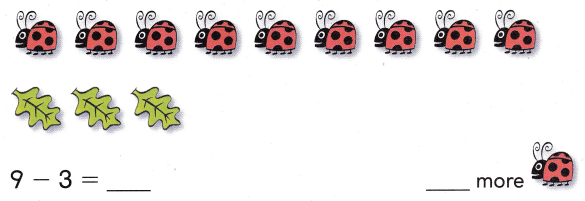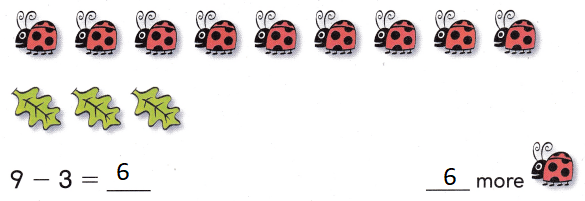Explanation:
9 – 3 = 6

Write or draw to solve.

Question 3.
Sam has 5 baseball bats and 3 baseballs. How many fewer baseballs does Sam have?
____ fewer baseballs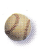Explanation:
Sam has 5 baseball bats and 3 baseballs.
So 5 – 3 = 2.
2 fewer baseballs does Sam have

Question 4.
H.O.T. Jill has 2 more cats than she has dogs. How many fewer dogs does Jill have?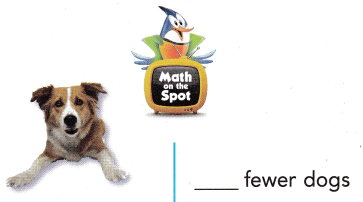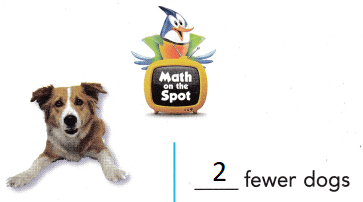Explanation:
4 – 2 = 2
so, 2 fewer dogs that Jill has

Question 5.
Multi-Step Kim has 6 plates and 4 cups. She needs 3 of each on the table. How many fewer plates and cups does she need on the table?
___ fewer plates
___ fewer cups
0 fewer plates
2 fewer cups
Explanation:
6 – 0 = 6
6 – 4 = 2
0 fewer plates
2 fewer cups she need on the tableQuestion 6.
Display How many moreare there?
(A) 2 more
(B) 5 more
(C) 3 more
Explanation:
5 – 3 = 2
2 more crows

Question 7.
Multi-Step Sid has 5 blue cars and 2 red cars. Ted has 4 cars. How many more cars does Sid have than Ted?
(A) 3 more
(B) 1 more
(C) 2 more
Explanation:
Sid has 5 blue cars and 2 red cars. So Sid has Total 5 +2= 7 cars
Ted has 4 cars.
7 – 4 =3 more cars does Sid have than Ted

Question 8.
Texas Test Prep How many fewer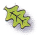are there?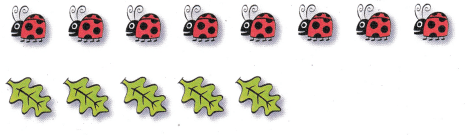(A) 2 fewer
(B) 3 fewer
(C) 5 fewer
Explanation:
8 – 5 = 3
3 lady bugs are there more
3 leaves are fewer

Take Home Activity

• Show your child two groups of up to 10 pennies. ‘ Have your child match them to see how many more or how many fewer, and then write a subtraction sentence to show the problem.
Explanation:
6 – 4 = 2
4 – 6 = 2
3 – 7 = 4
4 – 3 = 1

### Texas Go Math Grade 1 Lesson 5.4 Homework and Practice Answer Key

Subtraction to Compare

Question 1.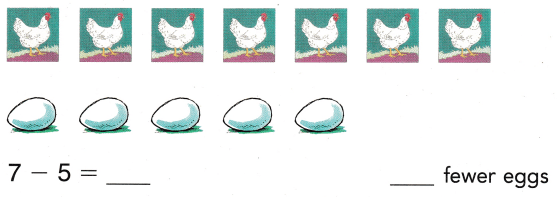Explanation:
7 – 5 = 2
2 hens are more

Question 2.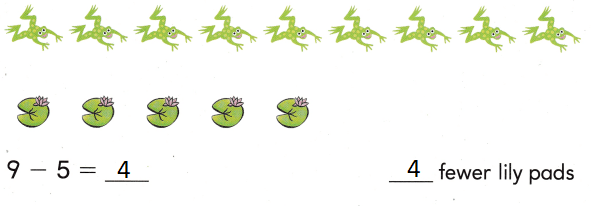Explanation:
9 – 5 = 4
4 frogs are there

Problem Solving

Write or draw to solve.

Question 3.
Ana has 4 pots and 7 seeds. How many fewer pots does Ana have?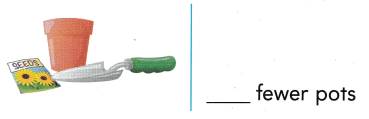Explanation:
7  – 4 = 3
3 fewer pots that Ana have

Texas Test Prep

Lesson Check

Question 4.
How many more are there?
(A) 6 more
(B) 1 more
(C) 5 more
Explanation:
1 more monkey is there

Question 5.
Jose has 3 fewer pants than he has shirts. How many more shirts does Jose have?
(A) 3 more shirts
(B) 5 more shirts
(C) 1 more shirt
Explanation:
Jose has 3 fewer pants than he has shirts. that means he has 3 more shirts than pants

Question 6.
Multi-Step Rod has 3 red marbles and 4 blue marbles. Kai has 6 marbles. How many more marbles does Rod have than Kai?
(A) 3
(B) 2
(C) 1
Explanation:
Rod has 3 red marbles and 4 blue marbles. So Rod has total 3 + 4 = 7 marbles.
Kai has 6 marbles.
7 – 6 = 1 more marbles does Rod have than Kai

Question 7.
How many fewerare there?(A) 5 fewer
(B) 6 fewer
(C) 7 fewer
6 fewerare there